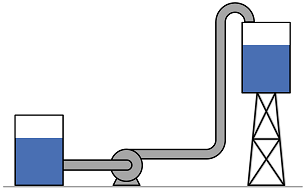# Summary

This article presents the method to convert between pressure and head for several common unit sets. Head relates the pressure of a fluid to the height of a column of that fluid which would produce an equivalent static pressure at its base. It is particularly useful for the specification of pumps as it provides a measure of pressure as it is independent of fluid density.

# Definitions

 : Gravitational Acceleration : Head : Specific Gravity : Pressure : Density

# Introduction

The pressure of a fluid describes the force per unit area which the fluid exerts in every direction. The head of a fluid in a system describes the height of an equivalent column of the fluid under gravity that would be required to achieve that pressure. To convert between the two measures need to know the density or specific gravity of the fluid.# Conversion Formulae

## Calculation in Metric Units (kPa & m)

The below equations may be used to convert between head and pressure when those measures are in the metric units kPa and m. Gravity is measured in m/s2.

Conversion from head to pressure:

Conversion from pressure to head:

## Calculation in US Customary Units (psi & ft)

The below equations may be used to convert between head and pressure when those measures are in the metric units psi and ft. Gravity is measured in ft/s2.

Conversion from head to pressure:

Conversion from pressure to head:

Article Tags

# Subscribe to our mailing list

 Email Name